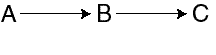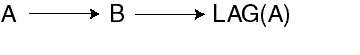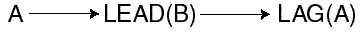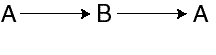Working with Models, 5 of 8

## Compiling a Model

When you finish writing the commands in a model, you can use the `COMPILE` command to compile the model. During compilation, `COMPILE` checks for format errors, so you can use `COMPILE` to help debug your code before running a model. If you do not use the `COMPILE` command before you run the model, then the model will be compiled automatically before it is solved.

When you compile a model, either by using the `COMPILE` command or by running the model, the model compiler examines each equation to determine whether the assignment target and each data source is a variable or a dimension value.

To resolve each name reference, the following procedure is used.

1. The dimensions in the `DIMENSION` commands are searched, in the order they are listed, to determine whether the name matches a dimension value of a listed dimension. The search concludes as soon as a match is found.
2. If the name does not match a value of a listed dimension, then the variables in the attached analytic workspaces are searched to find a match.

After resolving each name reference, the model compiler analyzes dependencies between the equations in the model. A dependence exists when the expression on the right-hand side of the equal sign in one equation refers to the assignment target of another equation. If an `=` command indirectly depends on itself as the result of the dependencies among equations, then a cyclic dependence exists between the equations.

The model compiler structures the equations into blocks and orders the equations within each block, and the blocks themselves, to reflect dependencies. The compiler can produce three types of solution blocks: simple blocks, step blocks, and simultaneous blocks.

### Simple Blocks

Simple blocks include equations that are independent of each other and equations that have dependencies on each other that are noncyclic.

If a block contains equations that solve for values A, B, and C, then a noncyclic dependence can be illustrated as shown below where the arrows indicate that A depends on B, and B depends on C.Text description of the illustration modsimp.gif

### Step Blocks

Step blocks include equations that have a cyclic dependence that is a one-way dimensional dependence. A dimensional dependence occurs when the data for the current dimension value depends on data from previous or later dimension values. The dimensional dependence is one way when the data depends on previous values only or later values only, but not both.

Dimensional dependence typically occurs over a time dimension. For example, it is common for a line item value to depend on the value of the same line item or a different line item in a previous time period. If a block contains equations that solve for values A and B, then a one-way dimensional dependence can be illustrated as shown in the figure below where arrows indicate that A depends on B, and B depends on the value of A from a previous time period.Text description of the illustration modstep.gif

### Simultaneous Blocks

Simultaneous blocks include equations that have a cyclic dependence that is other than one-way dimensional. The cyclic dependence may be two-way dimensional, or it may involve no dimensional qualifiers at all.

An example of a cyclic dependence that is two-way dimensional can be illustrated as shown below where the arrows indicate that A depends on the value of B from a future period, while B depends on the value of A from a previous period.Text description of the illustration modcyc1.gif

An example of a cyclic dependence that does not depend on any dimensional qualifiers can be illustrated as shown below where the arrows indicate that A depends on B and B depends on A.Text description of the illustration modcyc2.gif